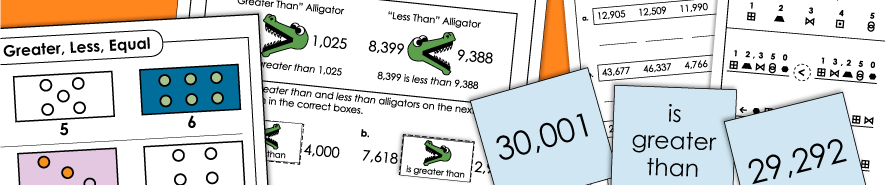# Greater Than / Less Than Worksheets

Worksheets for comparing numbers using greater than, less than and equal to symbols.  Difficulty levels vary from two-digit numbers up to six-digit numbers.  Worksheets include 'Greater Than Alligator' and 'Less Than Alligator' activities, as well as practice using the symbols <, >, and =.## Comparing and Ordering Numbers

1-Digit Numbers: Comparing

Compare single-digit numbers with mathematical symbols. Also includes worksheets on using words more and fewer. Illustrations on many worksheets require counting.

2-Digit Numbers: Comparing and Ordering

On this page, you'll find a large selection of worksheets on comparing and ordering 2-digit numbers. Teach students how to use the greater than, less than, and equal to math symbols. Includes a couple math games and learning centers, as well as worksheets.

3-Digit Numbers: Comparing and Ordering

This page has printables for ordering and comparing triple-digit numbers. Includes worksheets, task cards, a classroom games, a cut-and-glue alligator activity, and more.

4-Digit Numbers: Ordering and Comparing

Learn about "greater than", "less than", and "equal to" with these printable teaching resources. All include numbers up to four digits.

5-Digit Numbers: Comparing and Ordering

6-Digit Numbers: Comparing and Ordering

Use the mathematical symbols for "equal to," "less than," and "greater than" to compare numbers. Also, order numbers from least to greatest.

## Fractions, Money, Decimal Comparisons

Fractions: Comparing and Ordering

Decimals: Comparing and Ordering

On these printables, pupils will use the symbols <, >, and = to compare decimal values.

Money: Comparing

Compare pairs of money amounts. (example: \$1.56 < \$1.46) Includes worksheets and a math card game learning center.

Place Value

Here's our main place value page, which has a variety of resources that can help students learn about digit values, place value blocks, reading numbers, and expanded form.

Rounding

This set of teaching resources will help students learn about rounding to the nearest ten, hundred, thousand, tenth, and hundredth.

Inequalities

This index page features middle-school level worksheets on inequalities.